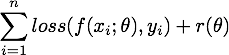18

6

8

5

# EmpiricalRisks

This Julia package provides a collection of predictors and loss functions, mainly to support the implementation of (regularized) empirical risk minimization methods.

Currently, the following higher-level packages are depending on EmpiricalRisks:

• Regression: solving moderate-size problem using conventional optimization techniques.
• SGDOptim: solving large-scale problem using stochastic gradient descent or its variants.

## Overview

This package provides basic components for implementing regularized empirical risk minimization:• Prediction models `u = f(x; θ)`

• [x] linear prediction
• [x] affine prediction
• [x] multivariate linear prediction
• [x] multivariate affine prediction
• Loss functions `loss(u, y)`

• [x] squared loss
• [x] absolute loss
• [x] quantile loss
• [x] huber loss
• [x] hinge loss
• [x] squared hinge loss
• [x] smoothed hinge loss
• [x] logistic loss
• [x] sum squared loss (for multivariate prediction)
• [x] multinomial logistic loss

Notes:

• For each loss function, we provide methods to compute the loss value, the derivative/gradient, or both (at the same time).
• For each (consistent) combination of loss function and prediction model (which together are referred to as a risk model), we provide methods to compute the total risk and the gradient w.r.t. the parameter.

• Regularizers
• [x] squared L2

• [x] L1

• [x] elastic net (L1 + squared L2)

Notes:

• For each regularizer, we provide methods to evaluate the regularization value, the gradient, and the proximal operator.

Remarks: All functions in this package are carefully optimized and tested.

## Documentation

Here is the Detailed Documentation.

04/16/2015

3 months ago

83 commits